# RD Sharma Solutions for Class 8 Maths Chapter 1 - Rational Numbers Exercise 1.7

In Exercise 1.7, we shall discuss problems based on the division of rational numbers and their properties. This set of solutions are prepared by our expert tutor team to help students understand the fundamentals easily. Solutions for RD Sharma Class 8 Maths Exercise 1.7 Chapter 1, Rational Numbers are provided here. Students can download from the links given below.

## Download PDF of RD Sharma Solutions for Class 8 Maths Exercise 1.7 Chapter 1 Rational Numbers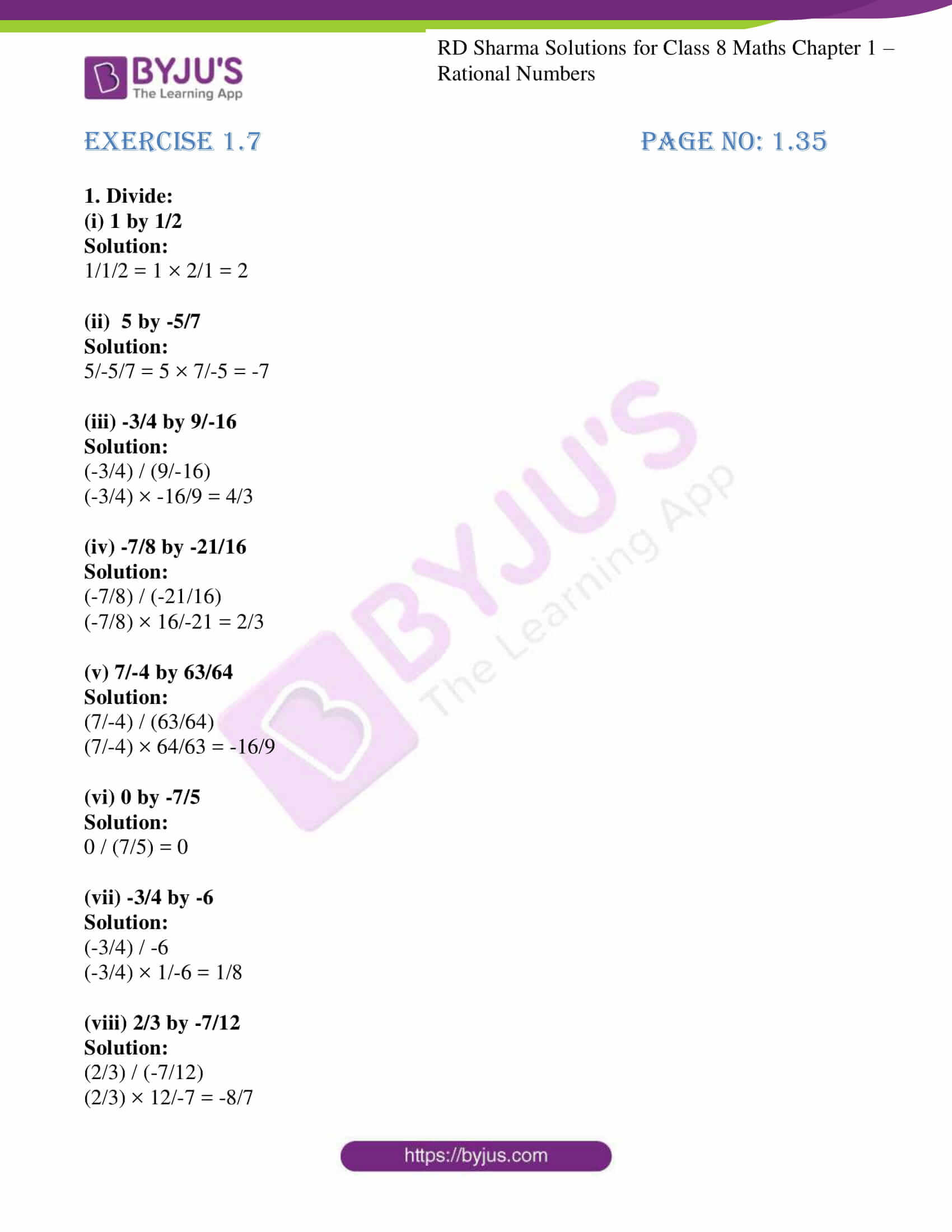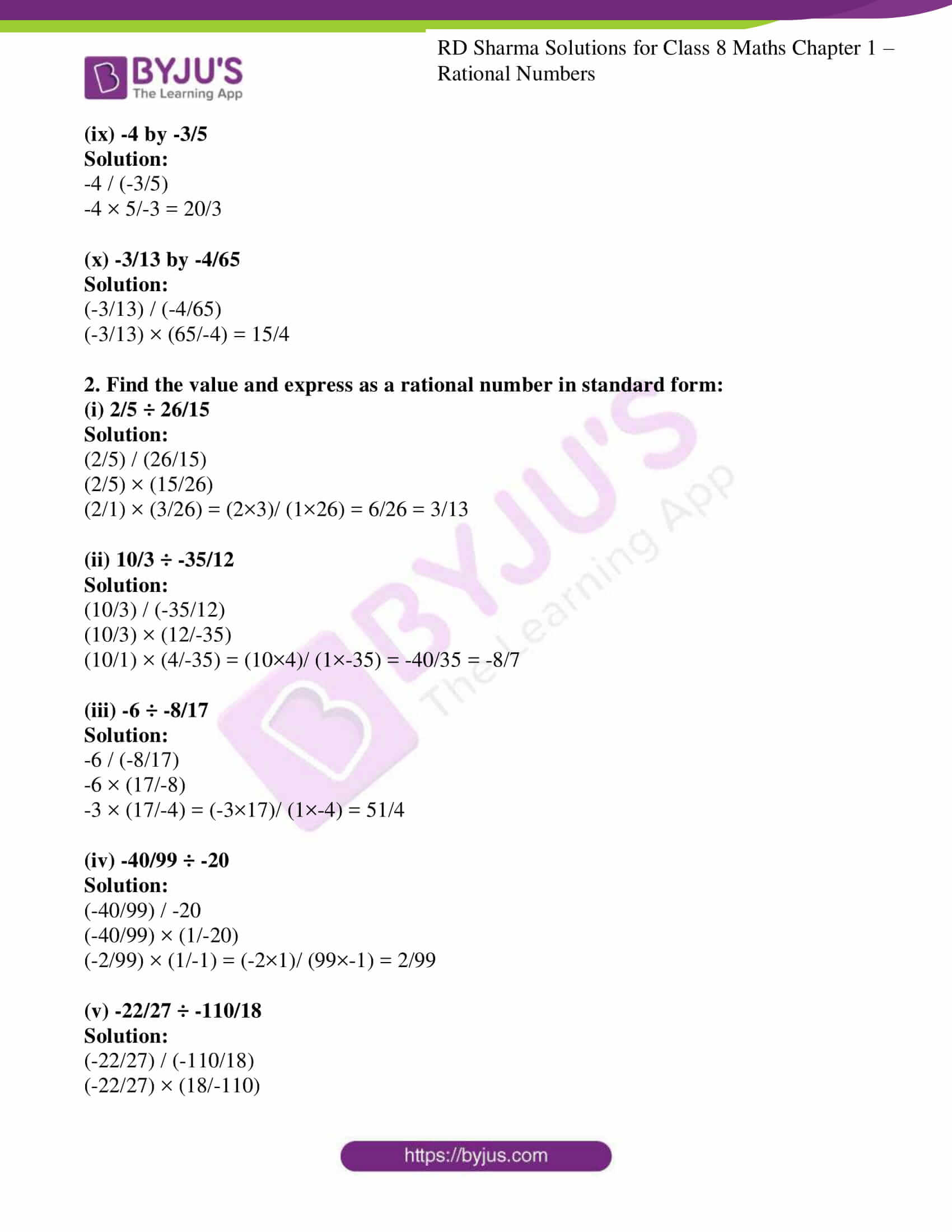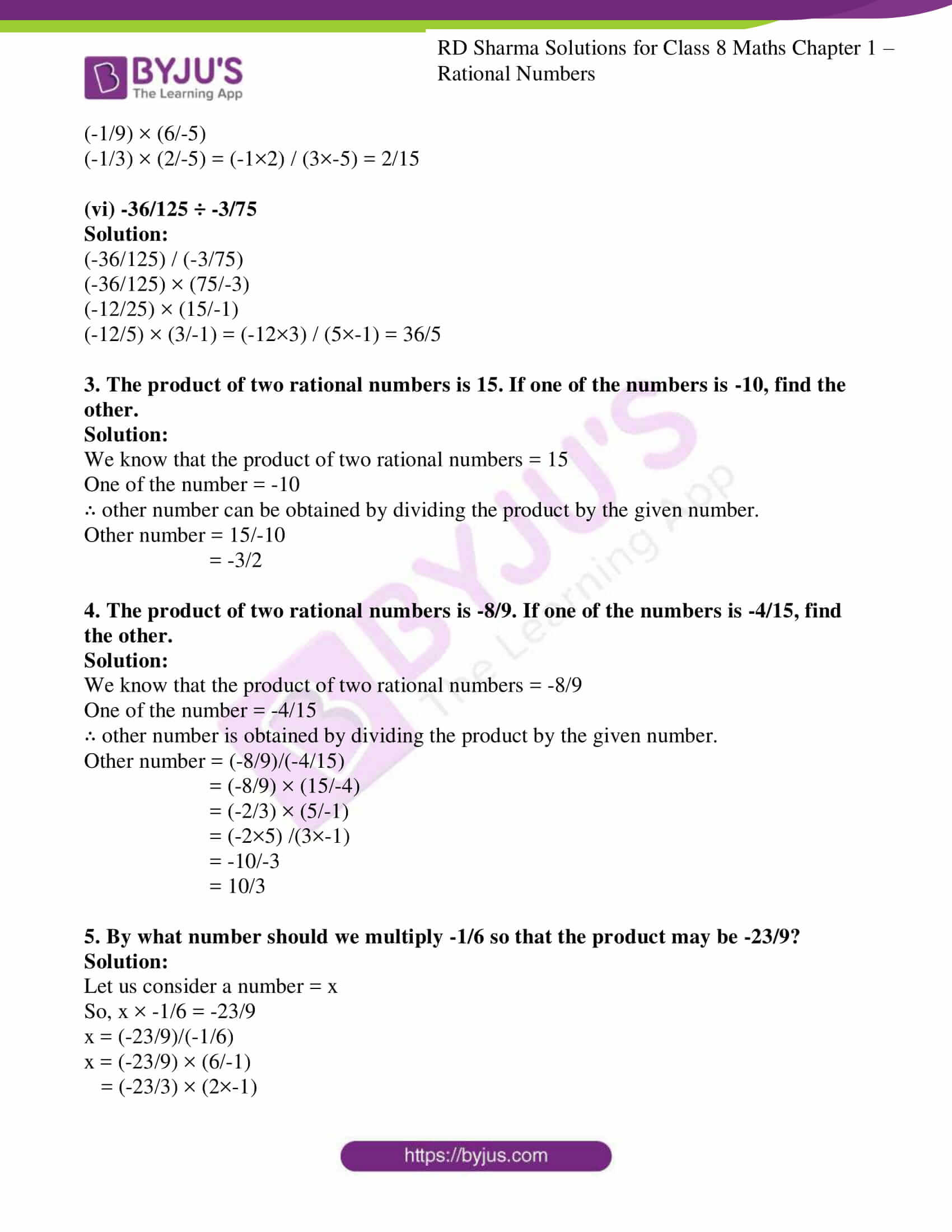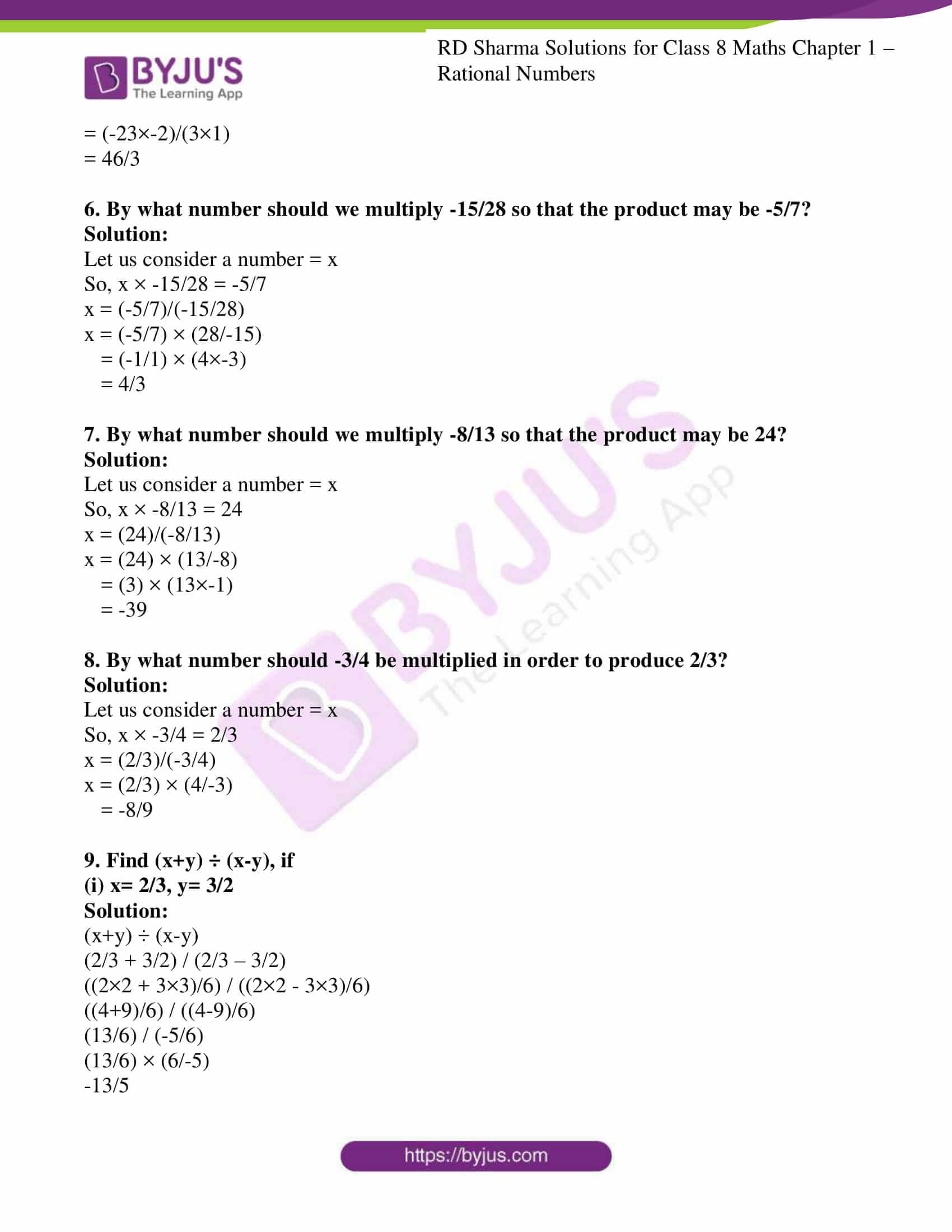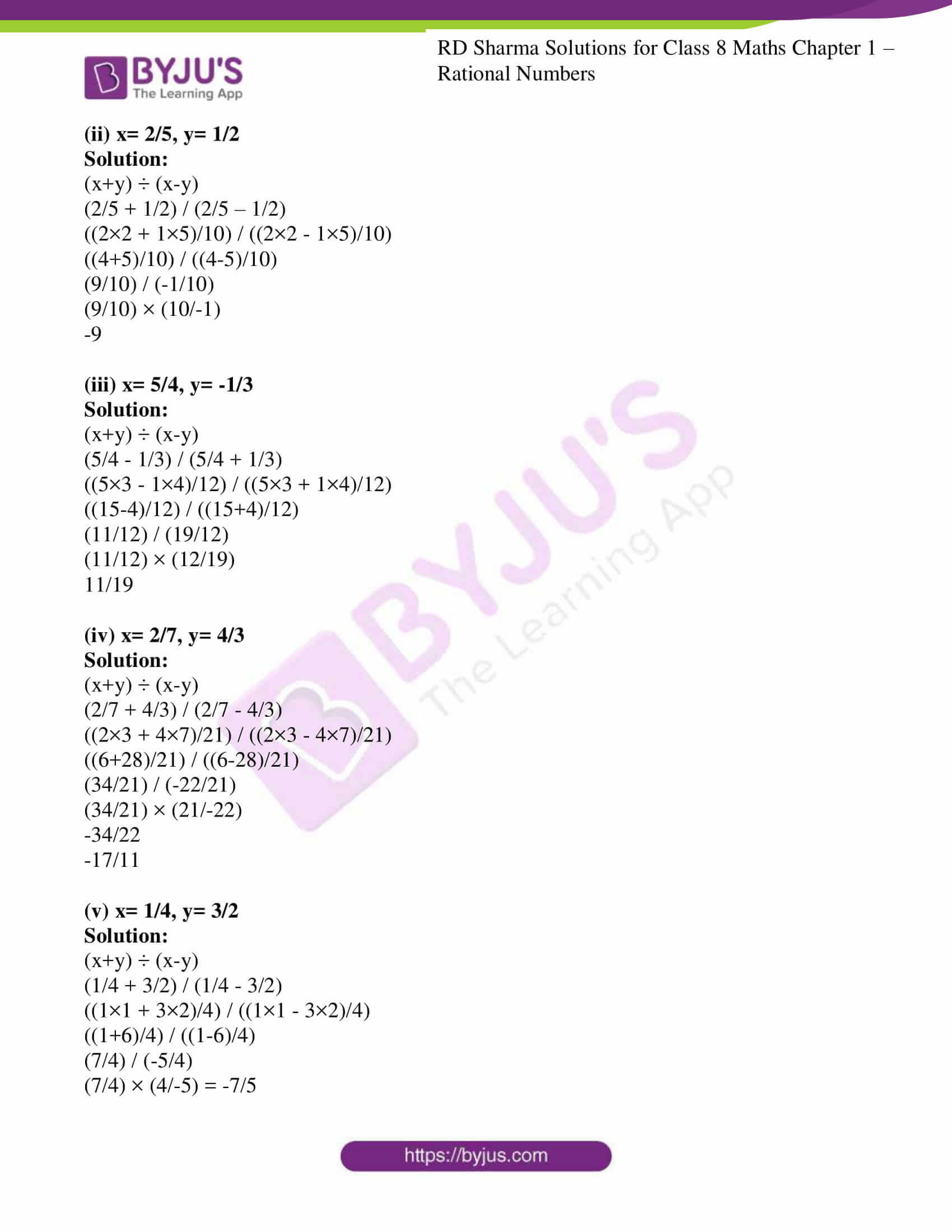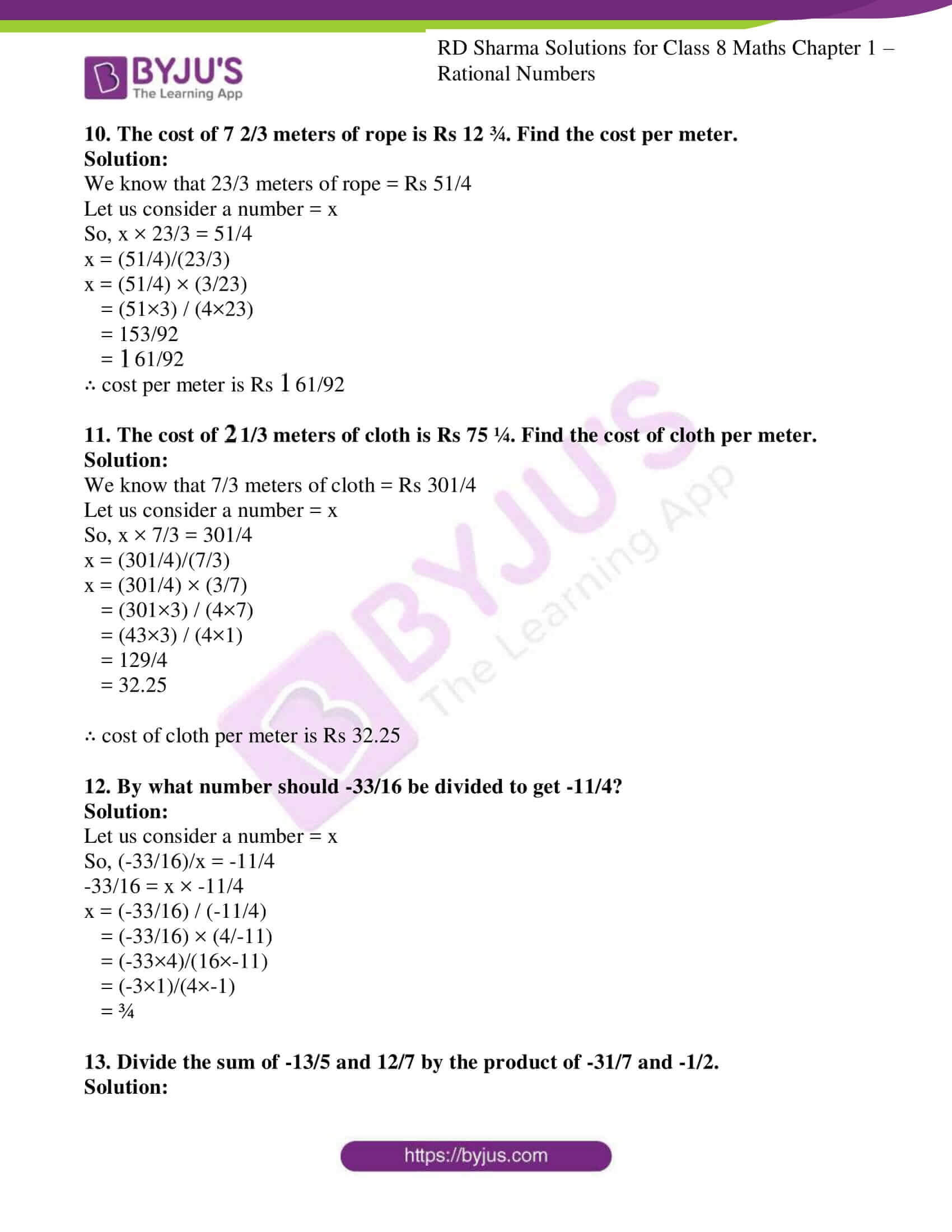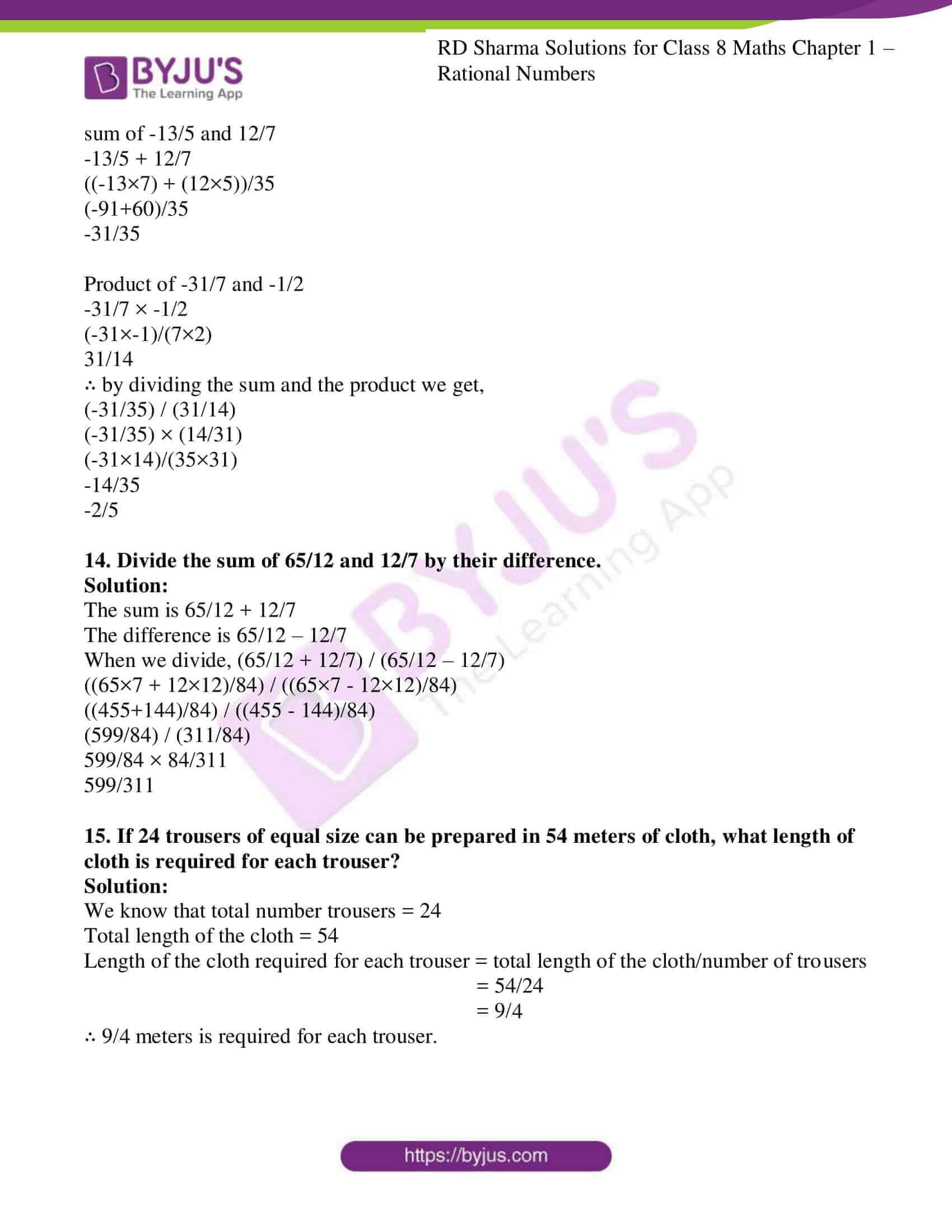### Access Answers to RD Sharma Solutions for Class 8 Maths Exercise 1.7 Chapter 1 Rational Numbers

1. Divide:

(i) 1 by 1/2

Solution:

1/1/2 = 1 × 2/1 = 2

(ii) 5 by -5/7

Solution:

5/-5/7 = 5 × 7/-5 = -7

(iii) -3/4 by 9/-16

Solution:

(-3/4) / (9/-16)

(-3/4) × -16/9 = 4/3

(iv) -7/8 by -21/16

Solution:

(-7/8) / (-21/16)

(-7/8) × 16/-21 = 2/3

(v) 7/-4 by 63/64

Solution:

(7/-4) / (63/64)

(7/-4) × 64/63 = -16/9

(vi) 0 by -7/5

Solution:

0 / (7/5) = 0

(vii) -3/4 by -6

Solution:

(-3/4) / -6

(-3/4) × 1/-6 = 1/8

(viii) 2/3 by -7/12

Solution:

(2/3) / (-7/12)

(2/3) × 12/-7 = -8/7

(ix) -4 by -3/5

Solution:

-4 / (-3/5)

-4 × 5/-3 = 20/3

(x) -3/13 by -4/65

Solution:

(-3/13) / (-4/65)

(-3/13) × (65/-4) = 15/4

2. Find the value and express as a rational number in standard form:

(i) 2/5 ÷ 26/15

Solution:

(2/5) / (26/15)

(2/5) × (15/26)

(2/1) × (3/26) = (2×3)/ (1×26) = 6/26 = 3/13

(ii) 10/3 ÷ -35/12

Solution:

(10/3) / (-35/12)

(10/3) × (12/-35)

(10/1) × (4/-35) = (10×4)/ (1×-35) = -40/35 = -8/7

(iii) -6 ÷ -8/17

Solution:

-6 / (-8/17)

-6 × (17/-8)

-3 × (17/-4) = (-3×17)/ (1×-4) = 51/4

(iv) -40/99 ÷ -20

Solution:

(-40/99) / -20

(-40/99) × (1/-20)

(-2/99) × (1/-1) = (-2×1)/ (99×-1) = 2/99

(v) -22/27 ÷ -110/18

Solution:

(-22/27) / (-110/18)

(-22/27) × (18/-110)

(-1/9) × (6/-5)

(-1/3) × (2/-5) = (-1×2) / (3×-5) = 2/15

(vi) -36/125 ÷ -3/75

Solution:

(-36/125) / (-3/75)

(-36/125) × (75/-3)

(-12/25) × (15/-1)

(-12/5) × (3/-1) = (-12×3) / (5×-1) = 36/5

3. The product of two rational numbers is 15. If one of the numbers is -10, find the other.

Solution:

We know that the product of two rational numbers = 15

One of the number = -10

∴ other number can be obtained by dividing the product by the given number.

Other number = 15/-10

= -3/2

4. The product of two rational numbers is -8/9. If one of the numbers is -4/15, find the other.

Solution:

We know that the product of two rational numbers = -8/9

One of the number = -4/15

∴ other number is obtained by dividing the product by the given number.

Other number = (-8/9)/(-4/15)

= (-8/9) × (15/-4)

= (-2/3) × (5/-1)

= (-2×5) /(3×-1)

= -10/-3

= 10/3

5. By what number should we multiply -1/6 so that the product may be -23/9?

Solution:

Let us consider a number = x

So, x × -1/6 = -23/9

x = (-23/9)/(-1/6)

x = (-23/9) × (6/-1)

= (-23/3) × (2×-1)

= (-23×-2)/(3×1)

= 46/3

6. By what number should we multiply -15/28 so that the product may be -5/7?

Solution:

Let us consider a number = x

So, x × -15/28 = -5/7

x = (-5/7)/(-15/28)

x = (-5/7) × (28/-15)

= (-1/1) × (4×-3)

= 4/3

7. By what number should we multiply -8/13 so that the product may be 24?

Solution:

Let us consider a number = x

So, x × -8/13 = 24

x = (24)/(-8/13)

x = (24) × (13/-8)

= (3) × (13×-1)

= -39

8. By what number should -3/4 be multiplied in order to produce 2/3?

Solution:

Let us consider a number = x

So, x × -3/4 = 2/3

x = (2/3)/(-3/4)

x = (2/3) × (4/-3)

= -8/9

9. Find (x+y) ÷ (x-y), if

(i) x= 2/3, y= 3/2

Solution:

(x+y) ÷ (x-y)

(2/3 + 3/2) / (2/3 – 3/2)

((2×2 + 3×3)/6) / ((2×2 – 3×3)/6)

((4+9)/6) / ((4-9)/6)

(13/6) / (-5/6)

(13/6) × (6/-5)

-13/5

(ii) x= 2/5, y= 1/2

Solution:

(x+y) ÷ (x-y)

(2/5 + 1/2) / (2/5 – 1/2)

((2×2 + 1×5)/10) / ((2×2 – 1×5)/10)

((4+5)/10) / ((4-5)/10)

(9/10) / (-1/10)

(9/10) × (10/-1)

-9

(iii) x= 5/4, y= -1/3

Solution:

(x+y) ÷ (x-y)

(5/4 – 1/3) / (5/4 + 1/3)

((5×3 – 1×4)/12) / ((5×3 + 1×4)/12)

((15-4)/12) / ((15+4)/12)

(11/12) / (19/12)

(11/12) × (12/19)

11/19

(iv) x= 2/7, y= 4/3

Solution:

(x+y) ÷ (x-y)

(2/7 + 4/3) / (2/7 – 4/3)

((2×3 + 4×7)/21) / ((2×3 – 4×7)/21)

((6+28)/21) / ((6-28)/21)

(34/21) / (-22/21)

(34/21) × (21/-22)

-34/22

-17/11

(v) x= 1/4, y= 3/2

Solution:

(x+y) ÷ (x-y)

(1/4 + 3/2) / (1/4 – 3/2)

((1×1 + 3×2)/4) / ((1×1 – 3×2)/4)

((1+6)/4) / ((1-6)/4)

(7/4) / (-5/4)

(7/4) × (4/-5) = -7/5

10. The cost of

$$\begin{array}{l}7\frac{2}{3}\end{array}$$
meters of rope is Rs 12 ¾. Find the cost per meter.

Solution:

We know that 23/3 meters of rope = Rs 51/4

Let us consider a number = x

So, x × 23/3 = 51/4

x = (51/4)/(23/3)

x = (51/4) × (3/23)

= (51×3) / (4×23)

= 153/92

=

$$\begin{array}{l}1\frac{61}{92}\end{array}$$

∴ cost per meter is Rs

$$\begin{array}{l}1\frac{61}{92}\end{array}$$

11. The cost of

$$\begin{array}{l}2\frac{1}{3}\end{array}$$
meters of cloth is Rs 75 ¼. Find the cost of cloth per meter.

Solution:

We know that 7/3 meters of cloth = Rs 301/4

Let us consider a number = x

So, x × 7/3 = 301/4

x = (301/4)/(7/3)

x = (301/4) × (3/7)

= (301×3) / (4×7)

= (43×3) / (4×1)

= 129/4

= 32.25

∴ cost of cloth per meter is Rs 32.25

12. By what number should -33/16 be divided to get -11/4?

Solution:

Let us consider a number = x

So, (-33/16)/x = -11/4

-33/16 = x × -11/4

x = (-33/16) / (-11/4)

= (-33/16) × (4/-11)

= (-33×4)/(16×-11)

= (-3×1)/(4×-1)

= ¾

13. Divide the sum of -13/5 and 12/7 by the product of -31/7 and -1/2.

Solution:

sum of -13/5 and 12/7

-13/5 + 12/7

((-13×7) + (12×5))/35

(-91+60)/35

-31/35

Product of -31/7 and -1/2

-31/7 × -1/2

(-31×-1)/(7×2)

31/14

∴ by dividing the sum and the product we get,

(-31/35) / (31/14)

(-31/35) × (14/31)

(-31×14)/(35×31)

-14/35

-2/5

14. Divide the sum of 65/12 and 12/7 by their difference.

Solution:

The sum is 65/12 + 12/7

The difference is 65/12 – 12/7

When we divide, (65/12 + 12/7) / (65/12 – 12/7)

((65×7 + 12×12)/84) / ((65×7 – 12×12)/84)

((455+144)/84) / ((455 – 144)/84)

(599/84) / (311/84)

599/84 × 84/311

599/311

15. If 24 trousers of equal size can be prepared in 54 meters of cloth, what length of cloth is required for each trouser?

Solution:

We know that total number trousers = 24

Total length of the cloth = 54

Length of the cloth required for each trouser = total length of the cloth/number of trousers

= 54/24

= 9/4

∴ 9/4 meters is required for each trouser.

## RD Sharma Solutions for Class 8 Maths Exercise 1.7 Chapter 1 Rational Numbers

Class 8 Maths Chapter 1 Rational Numbers Exercise 1.7 is based on the division of rational numbers. To facilitate easy learning and understanding of concepts download free RD Sharma Solutions of Chapter 1 in PDF format, which provides answers to all the questions. Practising as many times as possible helps students in building time management skills and also boosts the confidence level to achieve high marks.